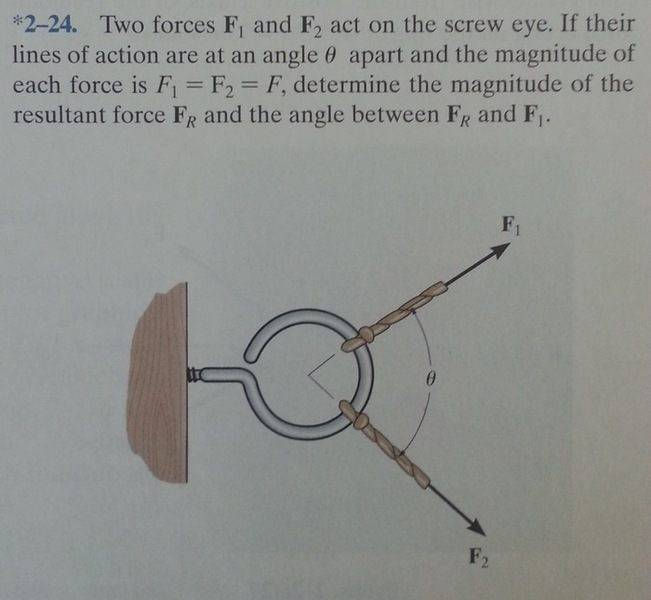# Engineering Statics Question

## Homework Statement## Homework Equations

F^2 (resultant ) = F1^2 + F2^2 -2F1F2cos(theta)

## The Attempt at a Solution

i calculated the magnitude using the equation above and it ended up being :

F (resultant) = F sqrt(2-2cos(theta))

i dont know how to solve for the angle.

## Answers and Replies

Nathanael
Homework Helper
Well in this particular case you can use symmetry (the magnitude of the forces are the same) to find it out.

In general, you could find the angle between two angles by solving for ##\phi## in this equation: $\vec a\cdot \vec b=|a||b|cos\phi$
(Of course, you would want to use some coordinate system to find ##\vec a\cdot \vec b##)

So in this case we say it's theta/2?

Nathanael
Homework Helper
So in this case we say it's theta/2?
Yep